Comenius University,

Faculty of Mathematics, Physics and Informatics,

Deartment of Computer Graphics

Procedural Textures Created on Cellular Automata

Peter Borovsky

Bratislava,                                                                                                                            2003

Slovakia

# Keywords

procedural texture, texture synthesis, cellular automaton, noise

# Purpose

This document is written as rigorous thesis in Computer Graphics applied at the Department of Computer Graphics, FMFI UK. We want to show reader our view on texture synthesis. We are generating 2D images with famous procedural texture algorithms as well as some we invented ad hoc. In this document cellular Automata (CA) are chosen as a tool for texture synthesis. Much of our work is available in digital form. There are our programs, their sources and a little more accompanied to this hardcopy.

Text and all figures in the document are property of Peter Borovský.

# Style

We did not want to overload reader with definitions and theorem proofs, therefore we choose less formal style of written text. Important term introduced first time is written in italics. We expect reader is acknowledged with some part of the computer graphics.

Document is divided into 6 sections. This is the introducting one. First section contains our categorization of texture synthesis techiques. Second section gives an overview of texture synthesis applications. Then comes short introduction to cellular automata. Our study of CA follows in section 4 and 5. Appendices are marked as section 6.

# Introduction

Texture synthesis, as well as overall computer graphics, joins many aspects of art, natural sciences and engineering. There is a theory background and, on the other side, there are practical applications helping users to enjoy the world of artificial images.

We assume reader is acknowledged with basics of texture synthesis. Anyway, we will mention some facts as for beginners.

In our work, we are not interested in geometry. We are not interested in hardware limitations. We are concerned in creating artificial patterns. Although they are computer-made, they resemble natural phenomena. Mammalian coats, marble, wooden textures and many others can be made using procedural techniques.

Graphic artists have two alternatives how to get texture:

(a)   Capture data from real world

(b)   Use procedural texture

Advantage of using procedural techniques is in easy parameterization. Procedural texture can be defined through a set of parameters suitable for particular application, whereas captured data are restricted in their substance. It is harder to change captured texture rather than change one parameter of the procedural shader.

# 1. Texture Synthesis Techniques

Term texture was first defined in 1974, when Edwin E. Catmull applied colors on parametric surface. Many definitions have been written since then. We do not want to define word texture precisely, because it became so popular that the meaning is clear. See [Ebert94] for detailed discussion.

In this paper, 2D textures are considered. Space, where texture is defined, is called texture domain (sometimes also texture space). Texture element is called texel.

We are not interested in texture mapping, which is a process of applying texture to an object.

Seamless texture is the one, which can be repeatedly tiled without seeing significant changes on tile borders.

According to topology, we are using two types of texture domains:

·         rectangle

·         torus

Rectangle is common 2D lattice. Torus is a rectangle where bordering texels are neighbouring with their counterpart (top/bottom, left/right). Toruses are used for generating seamless textures.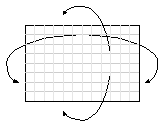Figure 1. Torus as a texture domain

In the rendering pipeline, software unit called shader is responsible for texturing.

Shaders are divided into 2 categories:

Vertex shader specifies color of a point on 3D object. Geometric data from vertexes on a polygon, where the point lies in, are passed to the input of vertex shader. Pixel shader takes place in the last stage of the rendering pipeline: rasterization. While the object is rasterized, pixel shader is evoked for each pixel. 2D viewport coordinates are most essential to the pixel shader input. Output can be used as any additional data. Various two-dimensional effects are gained by pixel shaders.

Texture synthesis is a process independent on the rendering pipeline. Many different procedural techniques arose in the past. Following text is not complete overview or introduction. We are going to briefly describe famous techniques, divided into 4 categories, and also our contributions with regard to further ideas.

## Explicit Functions

One dimensional explicit function f is function writable in the form

Equation(1)                             f(x) = formula(x)

Explicit functions are solid base for pretty looking continuous phenomena. Even sine synthesis yields satisfactory results in 1D.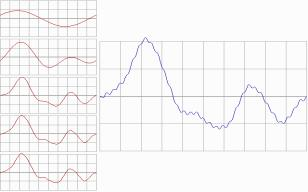Figure 2. Sine synthesis. Functional sequence on the left, last step on the right.

In the above figure, there is sine synthesis sketched. It is possible to use it for imitating mountain range profile. J. F. Blinn published 2D texturing technique based on Fourier synthesis in 1976. Anyone can play with explicit functions and get own results in one or two dimensions.

We gathered that creating textures from explicit functions in 2D is more complex than in 1D. See [boro00] for our attempts to get natural-looking patterns.

Texture synthesis as a creative process is sometimes understood like playing with explicit functions and geometry to shape desired pattern. This approach is often called magic with numbers. Steven Worley in [Ebert94] writes about toolbox functions. These are set of functions used by a graphic artist, experienced in geometry, to form the pattern. Textures like detailed honeycomb can be created this way.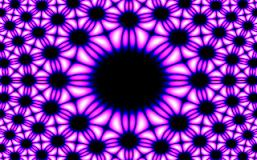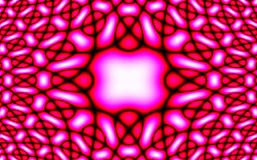Figure 3. Formulas of type sin( f(x), g(y) ) were used to get these patterns.

We had played with goniometric functions in raster. Two sample images are shown in the figure above. Both pictures have 320x200 texels. Program generating these pictures made one pass throughout whole texture space and defined a color from palette in each texel. Even both patterns can be described as surfaces defined by explicit functions, we visualized them with implicit algorithm: each point in raster was inquired for the value. This way we could also visualized any implicit surface, i.e. surface defined by the equation below.

Equation (2).                           f(x) = 0

We now want to point out complexity of texture generation. Under the term texture generation we mean a process of running texture synthesis program on hardware. If program generating textures on Figure 3 runs on one processor, it will need to perform 64000 computations on different input data. If we had parallel machine, program can do 64000 computations at once (Simple Instruction Multiple Data; SIMD principle). Anyway, it still requires the same computational time, because the algorithm runs in one step, in which Equation (1) is computed per texel. Solving this equation is atomic process, in general.

Parallel machines are more suitable for problems of greater time complexity, where evolution of texel value is necessary.

## Fractals

Fractals are very popular example of procedural modelling. Since there were many books published on this topic, we suppose reader is acknowledged with fractal geometry. In [Ebert94], fractal shields are discussed as the application suitable for terrain simulation. Hundred years ago, diagrams like von Koch’s snowflake were studied. In the 1970’s, Benoit Mandelbrot published his work. His name is linked with the famous set in 2D plane.

Dragon curve or von Koch’s snowflake are examples of hardly rasterized patterns. They consist of miscellaneously angled links in planar area. Vector graphics (e.g. turtle graphics) is needed for proper visualization.

Anyway, there are fractals with raster nature. For instance, mid-point displacement algorithm also known as diamond algorithm can be easily modified for grid of size 2nx2n.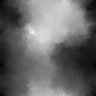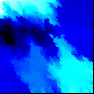Figure 4. Two sample outputs of modified diamond algorithm

Original diamond algorithm is well described in [diamond]. Purpose is to generate random 3D shield resembling mountain. It relies on dynamically constructed grid.

Our slightly modified diamond algorithm runs on static grid, which is a rectangular lattice. We implemented the algorithm in 2D, using colour instead of height. We had to take care of the grid size and stopping of the recursion. Hence, most suitable grid size is 2nx2n for n steps. Results of our diamond algorithm are shown above.

## Noises

It can be said, that much of randomness in procedural modelling is due to involving some noise function into the algorithm. First person revealing power of noises in raster was Ken Perlin. He set a lattice of size n´m and assigned random values to each node. To get noise value in arbitrary point in the plane, linear combination of values from 4 nodes nearest to it are interpolated. This is called two-dimensional Perlin noise.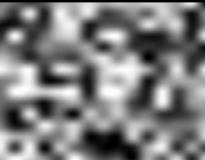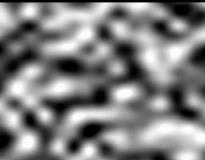Figure 5. Perlin noise on 15x15 grid. Bicubic interpolation on the left, compound bilinear on the right.

In the figure above reader can see two exapmles of Perlin noises. They differ in the interpolation used. If we displayed only 15x15 lattice defining the Perlin noise (shrunk so no blank spaces left there), just 2D white noise would be seen.

Definition of 2D noise consists of three requirements:

(1)   Noise is statistically invariant according to translation

(2)   Noise is statistically invariant according to rotation

(3)   Noise is chaotic (sharp frequency spectrum in Fourier domain)

Perlin noise satisfies all of them.

We are using noise of different nature than Perlin’s in our work. We call it averaged noise. Rather than defining it numerically, we describe its algorithm.

In the first step, rectangular grid is filled in with random values. In every next step, value for each texel is recomputed. It is set to average of 3x3 values from the neighbourhood (texel itself is also considered). Applying one step, nothing special would be seen. Applying more steps, interesting pattern will start to form.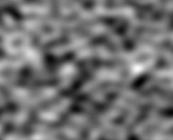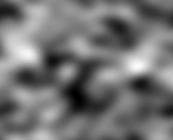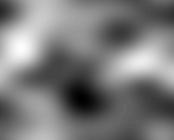Figure 6. Averaged Noise. Three stages of evolution were captured.

Figure above shows three stages (from left to right) of the noise evolution. Greyscale palette is used to highlight global minimum/maximum values on the grid. Bellow is the algorithm. It is written in rough form, for the implementation see Appendix A.

Input: grid, one texel is referenced by texel[x,y]

Step 0: for all meaningful x,y: texel[x,y] := random value

Step i: for all meaningful x,y: texel[x,y] := k * texel[x,y]

How to compute k in the step i:

k:= 0;

for i:= -1 to 1 do

for j:= -1 to 1 do

k := k + texel[x+i,y+j];

Understanding grid as a matrix, each step of the algorithm is convolution. This is the convolution matrix: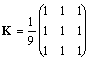It is an identity matrix multiplied by the constant. Hence, averaged noise satisfies noise function requirements (1) and (2). After stopping the algorithm in the step s, requirement (3) also satisfied. To make a clear proof, choice of s would depend on the grid size.

First step of the algorithm is 2D white noise. Whole system converges into stable pattern of an overall average value.

Matrix K can be altered. Then we get anisotropic effects. Anyway, sum of its members must be equal to 1 under all circumstances.

Let us compare 2D Perlin noise to averaged noise. Perlin noise requires mesh to be defined. Interpolation must be used for texels inside the mesh.

Averaged noise is any pattern from algorithm described above. Time of evolution define the scale; more steps are applied, greater the blurs are. It is due to isotropic diffussion. It can be used for procedural terrain generation (see Appendix A). However, noise alone is not suitable for generating mountain ranges, because averaged noise is defined implicitly. Matrix K changes only local properties of the pattern, it can not affect isotropism in global scale. On the other hand, mountain ranges are most simply defined as polylines. Polylines are explicit objects, whereas noise is implicit object. What about setting mountain range as polylines, and subsequently applying the averaged noise?

## Reaction-Diffusion

Although it is one of the youngest techniques used in texture synthesis, Alan Turing formalized reaction-diffusion (RD) already in 1952. It described chemical processes on cellular level in the field of embrional morphogenesis. Chemicals acting in the process were named morphogens. For detailed introduction into reaction-diffusion for computer graphics we refer reader to [Witkin91] and [Turk91].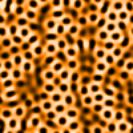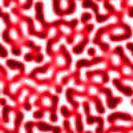Figure 7. Two outputs of the same RD system. Structural difference is due to different initial states.

RD can help to create mammalian coat patterns and other amazing images. Many articles including this topic appeared in 1990’s. We experimented with RD in [Boro00].

There were two main problems in RD according to computer graphics:

(1)   How to implement RD equation on computers

(2)   What equations are useful for computer graphics

Let us shortly discuss the first problem. In biology, RD is viewed as a space of cells interchanging their content. Cell is an irregular object. It interacts only with its closest neighbours.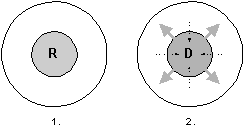Figure 8. Reaction and Diffusion.

There are 2 phases of RD step sketched in Figure 8. First is reaction, second one is diffusion. Diffusion is the only mechanism how can cell communicate with others.

In computer graphics, most effort was taken on efficient cell communication. Simplest RD systems were rectangular lattices where cells performed diffusion in parallel. Cell had 4 or 8 neighbours in this case. Other cell space topologies were also studied. Very useful was an idea of attaching cell space to 3D model and simulation of RD directly on that model. There are lot of papers considering texture synthesis on arbitrary surfaces using any reasonable procedural technique, now.

# 2. Texture Synthesis Applications

We overviewed 4 famous classes of procedural textures in the previous section. There is lot of software applications for each technique as well as systems combining them. Standalone authoring tools allowing to create user-defined procedural textures became popular, recently. They usually output finalized texture in some standart picture format. Our work can be considered as a development of such authoring tool.

However, we must be interested in what are the other possibilities. Let us recall some ideas relevant to texture synthesis.

## Cellular Automaton Projects

There are many scientific groups solving problems on cellular automaton. These are efficiently parallelized virtual lattices suitable for the field of computer simulation, in which procedural texture creation belongs to.

Before fast hardware shading, there were several renderers capable of software shading. Shaders were incorporated into the rendering pipeline, making users dependent on particular system. However, shading process usually consisted of simple texture layering by the mid-nineties. There was a possibility to import own textures. With increasing importance of texture synthesis, shaders began to be independent ([Porter98], Blue Moon Rendering Tools, etc.).

## Plugin Systems

There are cutting-edge rendering and modelling systems offering comfort of modularity (we mean plugin systems). One can write its own shader and experiment with texture creation. Importance of scripts (e.g. Python) and high-level languages (C/C++) arises. Implementing an algorithm for different rendering systems at once is possible.

## Authoring tools for texture synthesis

Totally independent authoring tool for texture creators has one main advantage: graphic artist needs to know nothing about scene geometry. Flat textures are constructed, instead. Output of such system is exported to software/hardware renderer.

## CG

One of the market leaders in PC graphic cards machinery introduced shading language called “CG” in 2002. It is based on the well-known C language. We think that this could be the way of writing shaders in the future, because shader developer is not disturbed with hardware properties.

Reader can dispute, that there are other shading standards on the market. Yes, CG is not the only one. But it is a very demonstrative example of how important for the rendering pipeline shading process is.

What texture synthesis techniques are suitable to implement in CG? The answer should be: any. But the real question is: how much effort is needed for coding particular procedural texture? And the answer depends on:

·         Knowledge of CG

·         Decision of shader type (vertex/pixel)

·         Visualization (color texture/bump map/displacement/opacity/…)

·         Debugging tool

Current drawback of CG is that although it is declared as hardware independent, one has to have a particular hardware before running CG runtime. Otherwise, developing and debugging CG code could be unproductive.

# 3. Short Introduction to Cellular Automata

We describe cellular automata (CA) according to texture synthesis in this section. Reader would find more CA applications related to computer graphics in [Takai].

Cellular automaton is system consisting of:

·         Finite CA space

·         Initial state

·         Rules of evolution

Element of CA space is called cell. Each cell is in one of its states. Evolutional rule tells cell how to behave. Computation on cellular automaton starts at initial step, where initial states are assigned to cells. Next steps are computed using evolutional rules. They are same for each cell. Cellular automaton is SIMD (Simple Instruction Multiple Data) parallel system, indeed.

Common CA definition citated from [NIST]:

Definition: Usually a two-dimensional organization of simple finite state machines whose next state depends on their own state and the states of their eight closest neighbors. In general the machines may be arranged in meshes of higher or lower dimension, have larger neighborhoods, or be arbitrarily complex processors.

Note: Conway's game of Life is probably the most well known instance of a cellular automaton.

## Cellular Automaton as Parallel System

We said that cellular automaton is SIMD parallel system. Such systems are studied on parallel machines, which solve problems by dividing them into smaller pieces. On the other hand, we have distributed systems.

Distributed system is a net, where each node computes its part of the problem. Difference between parallel systems and distributed systems is that we have to know number of processors in the parallel machine, whereas distributed system can be dynamic net. Demonstrative example of distributed system is radio sample analysis for the SETI project [SETI@home]. Anyone having an access to the Internet can join.

# 4. Binary CA

Simplest instance of cellular automata are binary cellular automata. We wanted to know, what textures could this category yield. 4-neighbourhood binary CA was chosen for our experiments. Texture domain consists of rectangular lattice with torus topology (illustration on Figure 1), here. Cell is either in the state 0 or in the state 1.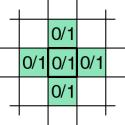Figure 9. Cell and its 4-neighbourhood in the binary CA

32 bits define program for the cell, because next state of the cell depends on its own state plus state of 4 neighbours. Hence, our binary CA defines 232 (4 294 967 296) programs. We wanted to know, how many of these programs define interesting patterns/animations.

We developed an application, which interprets arbitrary program (32 bits on input) on binary CA. White noise is set as the initial state. After interpreting program in each state, CA space is visualized: black color is associated to state 0, white color to state 1. Program interpretation is so fast, that the system visualization is fluent animation.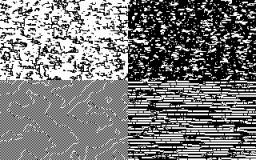Figure 10. Textures created by randomly generated programs on binary CA

Typing binary programs manually wasn’t very comprehensive, therefore we decided to generate the programs randomly. This method showed us surprising results: one can see amount of interesting patterns/animations between dull pixel formations! Even simple reaction-diffusion textures may be found there (see Figure 10 for sample outputs). We estimated, that about 1 from 5 randomly generated programs gives some attractive pattern. Of course, what is and wat is not attractive depends on the viewer’s attitude (more in [boro00]).

To sum up, animation on 4-neighbourhood binary CA can be specified by cell program and initial state. We write source code for cell program as 32bit vector. In the initial state, random binary is set to the cell. This method yields many binary animations. If we run the same program on large grid (40x40) with different initial states, we get statistically same animations. Choice of particular white noise does not influence the pattern/animation characteristics, it influences only the amount and positions of its typical local properties. Hence, all statistically same animations can be treated as one binary evolutional texture. In the other words, 32bit vector specifies any binary evolutional texture runable on our binary CA.

Source code for the cell program may be represented as 32bit number. Therefore, 4 294 967 296 binary evolutional textures are defined on our binary CA. Moreover, source code of the program can be created randomly.

# 5. Adding more complexity into binary CA

Averaged noise from Figure 6 cannot be defined on CA. More complexity is needed here. Thus we extended the power of binary CA by considering 6 floating-point variables instead of one binary. We get much more than binary textures, because 6 decimals specify more states than 1 bit. For example, all famous reaction-diffusion systems use no more than 6 variables per cell.

But, there was a question: how should the cell program look like? If we apply same method as in the previous section, we get very strange source code. It would consist of 26 x 5 x sizeof(decimal) x 8 bits , thus it would not be writable/readable. Additionally, no interpreter of such source code would be fast enough.

We specified language, called TxrLan in this document (abbreviation from Texture Language), as a solution more comfortable for a texture creator. It is language made of conditionals. Program is list of up to 6 rules – one rule for each variable. Rule defines next variable’s value. Rather than specify the language precisely, we demonstrate its nature on the example.

## Averaged noise implemented in TxrLan

Suppose convolution noise with this convolution matrix: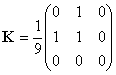The matrix defines anisotropic averaged noise. We need only one cell variable. TxrLan user has to decide, what pattern will start the evolution. In our solution, up to timestep 3, random value is assigned to the cell. Then, convolution is made by averaging the left, upper and the cell own variables.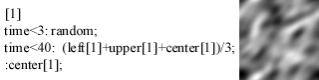Figure 11. Example of TXR LAN: code on the left, visualization on the right.

Source code in the above figure may be read line–by–line as follows:

This is rule for my 1st variable

if (time < 3) then variable := random,

else if (time < 40) variable := average of neighbours’ and my 1st variables,

else variable := my 1st variable

Function random outputs random floating-point value from interval á0,1ñ. Interpretation of exampled source code begins by a white noise. Blending of neighbouring values during the algorithm causes kind of wax melting effect. Difference to average noise as implemented in Appendix A is that this pattern is anisotropic. Resulting animation shows average noise shifting in downright direction. We did not study, if pattern in Figure 11 is equivalent to isotropic average noise.

## Discussion on TxrLan

No language is useful without practical applications. What are the applications suitable for TxrLan? Reader should know these:

·         Binary evolutional textures

·         Reaction-diffusion

·         Convolution noises

We know them as texture synthesis techniques. They can be viewed as numerical problems, too. TxrLan can help to solve lot of other problems ([boro00]).

There is another question: what is TxrLan not suitable for? We tried to implement diamond fractal algorithm, but the source code was too long, thus unreadable. We gathered that any phenomenon described explicitly is not suitable for TxrLan. Rather than theory, we introduce an example.

 Circle, radius 15    sqrt(sqr(x-20)+sqr(y-20)) < 15.5: 1; :0;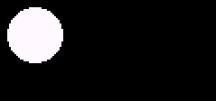Figure 12. Circle coded in TxrLan

Above is source code for one circle. Although all cells are running the same program, majority has the same state during the evolution. Why? There are two reasons: spatial and temporal.

Spatial

Let us freeze the time. Circle is an explicit object. Only inner cells specify it. Outer cells are not interesting. Another object can be defined outside the circle.

Temporal

Let us forget the space and consider one cell only. When this cell changes its state? Possibly in the first step, never after then.

We have no explanatory capabilities to precisely abstract the idea. We can just say, that circle (example of object explicitly defined in space and time) wastes power of TxrLan interpreter.

Let us introduce small analysis on what does it mean to implement program optimally on CA. We remark, that TxrLan is an instance of CA.

Suppose problem p formally defined on CA. Develop a program, which solves p on cellular automaton. Start the program. Call the situation in which cell is chaning its state one swap. Measure, how many swaps are during the program execution. Denote the result as M(p).

Observation:

Great value of M(p) indicates, that problem p is effectively solved on CA.

In our circle example, M(circle) = 177 (approximated as p ´ 7.52).

In the example from Figure 11, M(avenoise) = maxX x maxY x 40 (maxX, maxY specify grid size). Notice, that M(avenoise) is maximal possible. It proves, that program from Figure 11 solves averaged noise problem optimally.

Abstraction:

Problem p is optimally implemented on CA, if and only if M(p) = maximal possible.

This was our analysis. It looks fine, but we used language of computer graphics rather than parallel systems. Theory of parallel computing was based in [Jaja92]. It assumes that reader is educated in sequential programming. Our analysis was not influenced by sequential programming.

## Conclusion

We believe that reader understood our idea of joining CA and texture synthesis. If there is a demand, we will try to develop language more suitable for graphic artists than TxrLan. This language would be structural, i.e. it would allow to use variables as required. Source code would be far more readable.

We excuse for accuracy in this document. We wanted to describe the matter rather than formalize partial problems.

peter.borovsky@fractal.dam.fmph.uniba.sk

# 6. Appendices

This document is describing our work in the written form. Reading it is sufficient for people curious about procedural textures, CA or related topics. All examples in the document are static images. For closer understanding of dynamic phenomena, software applications and/or animations should be seen. Therefore we collected our experimental software applications and made it available onto accompanied CD.

All applications should run under Windows98/200/NT operating systems. Some of them where originally developed under MS-DOS.

For closer understanding, runable programs must be seen.

There are our source codes and executables available on the CD. There are also www pages and other materials related to the theme, which are properties of their authors.

## Appendix A

We described averaged noise in this paper. However, any algorithm description is not a definition. Therefore we print out following C++ source code. It is located at

sources \ averaged noise \ main.cpp

on the accompanied CD. Knowledge of C++ is necessary to understand the code. It was written with attention to readability.

Compiled application runs 8.5 seconds on Athlon XP 1600 CPU. It executes 50 convolution steps on 64000 texels. Hence, one texel value is recomputed in 0.00000265625 seconds. Most time consuming operation is texel visualization.

//---------------------------------------------------------------------------

/*

averaged noise definition

*/

/*

first_step()           makes 2D white noise.

step()                 performs one convolution step on pfSpace[][].

TfrmMain::show_space() visualizes one step.

Algorithm is triggered by TfrmMain::Trigger() function.

*/

#include <vcl.h>

#pragma hdrstop

#include "main.h"

//---------------------------------------------------------------------------

#pragma package(smart_init)

#pragma resource "*.dfm"

TfrmMain *frmMain;

#define MAX_X 320

#define MAX_Y 200

float pfSpace[MAX_X][MAX_Y];

float pfBackup[MAX_X][MAX_Y];

//---------------------------------------------------------------------------

first_step()

{

randomize;

for (int i = 0; i < MAX_X; i++)

{

for (int j = 0; j < MAX_Y; j++)

{

pfSpace[i][j] = rand() % 1000;

}

}

}

step()

{

// backup space

memcpy( pfBackup, pfSpace, MAX_X * MAX_Y * sizeof(float) );

// make lattice convolution

for (int x = 0; x < MAX_X; x++)

{

for (int y = 0; y < MAX_Y; y++)

{

// compute convolution for texel [x,y]

float f = 0;

for (int i = -1; i <= 1; i++)

{

for (int j = -1; j <= 1; j++)

{

f += pfBackup[(x+MAX_X+i) % MAX_X ][(y+MAX_Y+j) % MAX_Y ];

// rollover causes seamless effect

}

}

f /= 9; // 3x3 neighbourhood is considered

pfSpace[x][y] = f;

}

}

}

TfrmMain::show_space()

{

// get minimum/maximum from the space

float fMin = 100000;

float fMax = -1;

for (int x = 0; x < MAX_X; x++)

{

for (int y = 0; y < MAX_Y; y++)

{

if ( pfSpace[x][y] < fMin )

{

fMin = pfSpace[x][y];

}

else if ( pfSpace[x][y] > fMax )

{

fMax = pfSpace[x][y];

}

}

}

// get global disperse

float fDisp = fMax - fMin;

// show space

for (int x = 0; x < MAX_X; x++)

{

for (int y = 0; y < MAX_Y; y++)

{

float f = (pfSpace[x][y] - fMin) / fDisp;

Canvas->Pixels[x][y] = RGB( 255.0 * f, 255.0 * f, 255.0 * f);

}

}

}

//---------------------------------------------------------------------------

void __fastcall TfrmMain::Trigger(TObject *Sender)

{

// 1st step is setting of random values

first_step();

// do 50 steps of evolution

for (int i = 0; i < 50; i++)

{

step();

show_space();

lbStep->Caption = i+1;

Update();

}

}

//---------------------------------------------------------------------------

We used average noise in programs for generating earth relief procedurally. One of our executable can be found on the CD in

executables \ averaged noise\ relief

It is an old-fashioned computer game written in Borland Pascal environment under MS-DOS operating system. We encourage reader to run it, because there is a step–by–step procedural terrain generation animated well.

## Appendix B

TxrLan is interpreter of language we proposed in [Boro00]. Input is any text file with a cell program. Cell program consists of rules – one rule for one variable. Interpreter can be found on the CD in

First TxrLan interpreter was developed in 1999, stable version 1.6 was presented in 2000. Recently we upgraded the software for presentation purposes. It is located in

executables \ TxrLan

There are many example source codes. We encourage reader to play with them. Changing appropriate constant sometimes leads to unexpected and still interesting animation. Here follows samples. Source codes are on the left, outputs captured as static images are on the right. In the third sample only 2 from 3 morphogens are shown.

 chessboard, 8x8 px fields    mod(y,16) < 8:   mod(x,16) < 8: 1;   :0;   mod(x,16) >7: 1; :0;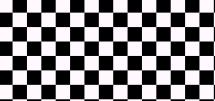Circle, radius 15    sqrt(sqr(x-20)+sqr(y-20)) < 15.5: 1; :0;Reaction-diffusion system, 3 morphogens    time<2: 4; :center+0.03125*(16-center*center)+0.25*(left+right+upper+lower-4*center);    time<2: 4; :center+0.03125*(center*(center-1)-center)+0.0625*(left+right+upper+lower-4*center);    time<2: 12+random*0.05-0.025; :center;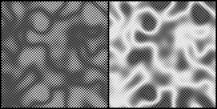## Appendix C

At [CG], we found a contribution of RD coded in CG. Interested reader would found the code on accompanied CD as

www \ RD in CG.htm

We do not print it out. The purpose of this appendix is to show a difference between shading language such as CG and language for texture synthesis. In a shading language program, much of an effort is taken on visualization. Our TxrLan does not care about visualization. Therefore, reaction-diffusion system can be coded in couple of lines as showed in Appendix B.

TxrLan has main disadvantage against CG in debugging. We tried to debug programs under construction by assigning special values to examined cells. It worked, but for huge source codes some user-friendly debugging is more convenient.

# References

[Ebert94]               D. S. Ebert, F. K. Musgrave, D. Peachey, K. Perlin, S. Worley: Texturing and Modeling: A Procedural Approach, Academic Press, Cambridge, 1994.

[Witkin91]             A. Witkin, M. Kass: Reaction-Diffusion Textures, in W. Sedeberg, editor: Computer Graphics (SIGGRAPH ’91 Proceedings), volume 25, pp. 299-308, 1991.

[Turk91]                G. Turk: Generating Textures on Arbitrary Surfaces Using Reaction-Diffusion, in W. Sedeberg, editor: Computer Graphics (SIGGRAPH ’91 Proceedings), volume 25, pp. 289-298, 1991.

[Jaja92]                  J. Jájá, An introduction to Parallel Algorithms, Addison-Wesley (ISBN 0-201-54856-9), 1992

[Talia00]                D. Talia: Cellular Processing Tools for High-Performance Simulation, in: IEEE Computer, volume 44, September 2000.

[Boro00]                P. Borovský, Procedurálne Textúry, Diploma thesis, MFF UK, 2000

[DarkTree]            Darkling Simulations: http://www.darksim.com

[Takai]                   Graphics Applications of Cellular Automata: http://madeira.cc.hokudai.ac.jp/RD/takai/automa.html

[NIST]                   Dictionary of Algorithms and Data Structures: http://www.nist.gov/dads/HTML/cellulartmtn.html

[SETI@home]      SETI@home experiment: http://setiathome.ssl.berkeley.edu/

[diamond]             Mid Point Displacement Algorithm: http://www.lighthouse3d.com/opengl/terrain/index.php3?mpd2